Follow

# Event Aggregation Methods

This article will be updated in the near future! In the meanwhile, if the following information is insufficient, contact support@flow-software.com.

Included in Flow are a number of aggregation methods which can give insight into Events in your Flow model

A measure that is configured with an event aggregation is still a time-based measure (i.e. it will still process periodically, according to the interval type that is configured for the measure), but it is able to give one information pertaining to an event.

To configure an event aggregation, drag an Event onto the interval in the Flow Zone for which you want to create the event aggregated measure.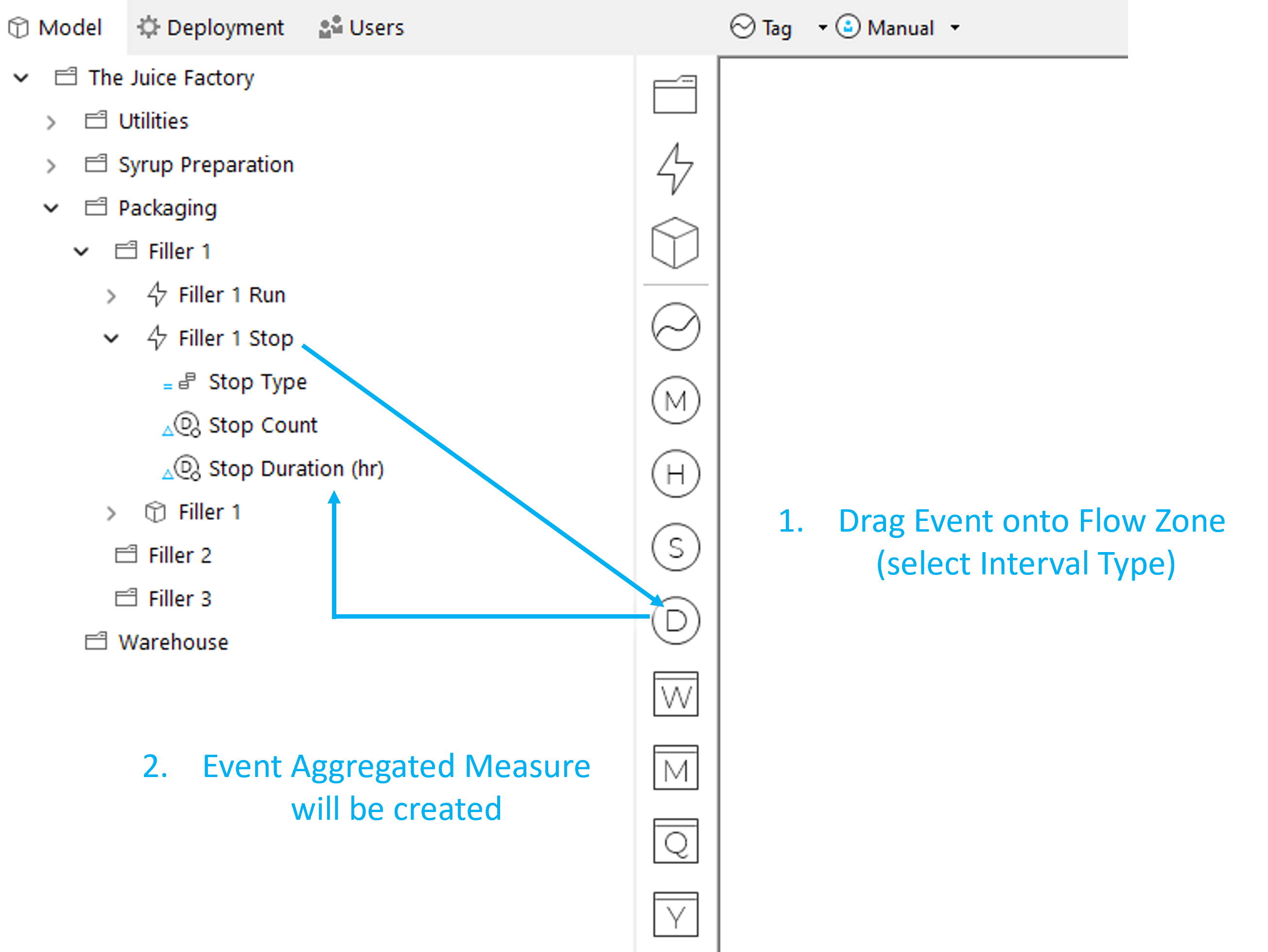The following Aggregation Methods are available and discussed below.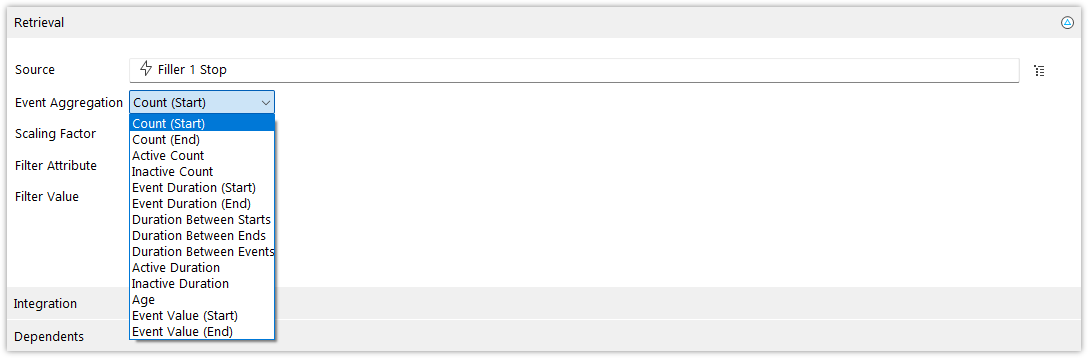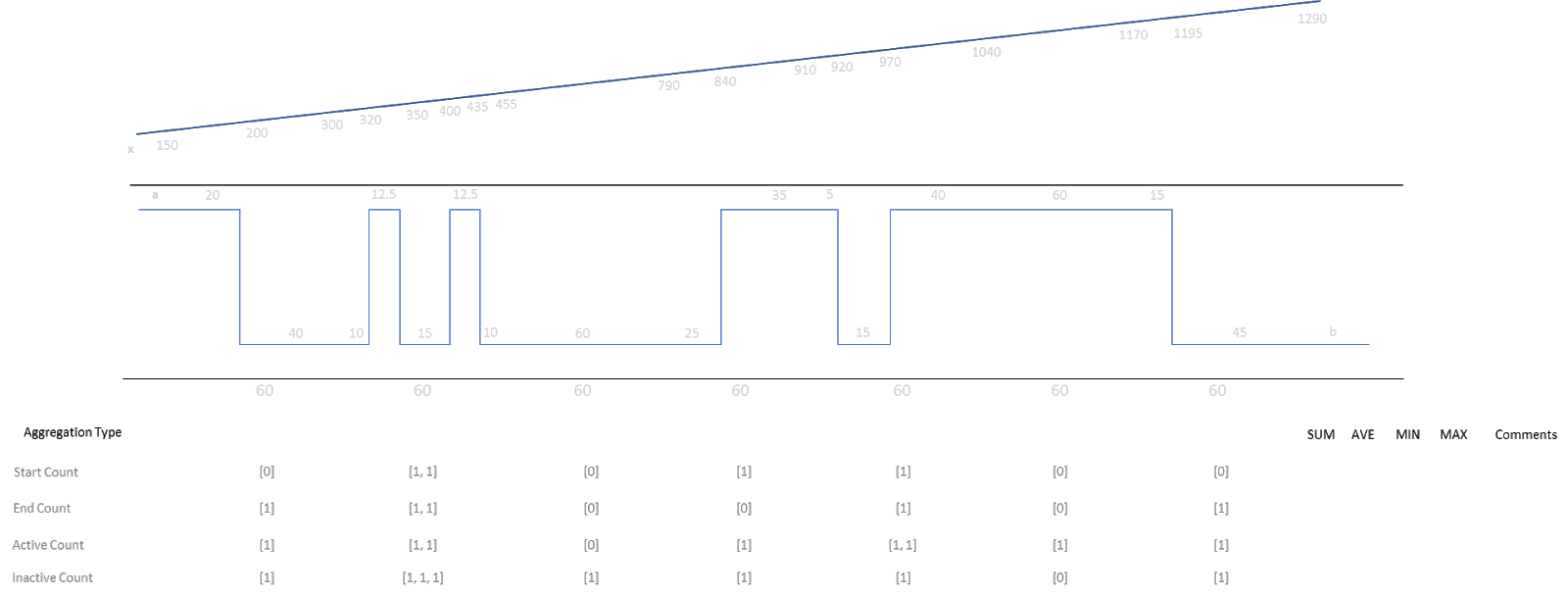### Start Count

In the interval for which the measure is processing, how many times did the event start, i.e. was its start trigger condition true?

### End Count

In the interval for which the measure is processing, how many times did the Event end, i.e. was its end condition true, or was there a new start trigger for an event that only has a start trigger and no end trigger configured.

### Active Count

In the interval for which the measure is processing, how many distinct times was the Event activated/started?

Of interest, it could be that there are no event starts in this interval, but that it was started in a previous interval and remained active through the interval, in which case the Active Count will be 1, while the Start Count will be 0.

### Inactive Count

In the interval for which the measure is processing, how many distinct times was the Event deactivated/ended?

Similar to the Active Count, it could be that there are no event ends in this interval, but it was ended in a previous interval, and remained inactive through the interval, in which case the Inactive Count will be 1, while the End Count will be 0.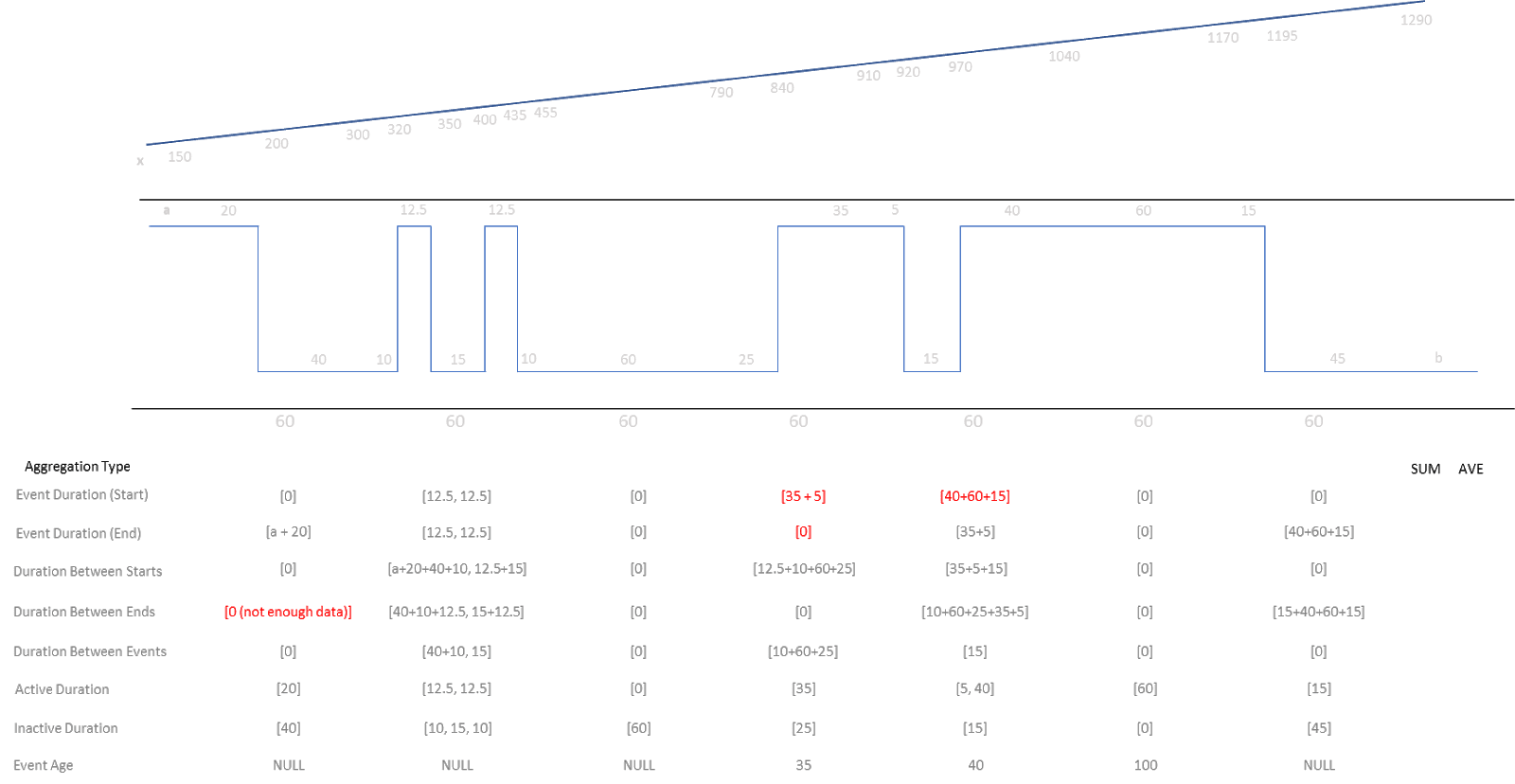The previous image helps to explain the following four event aggregation methods.

Unlike the previous four aggregation methods, which returned a scalar value, the following 7 methods (excluding Event Age) will return a set of values per event period in the interval. Thus, one would need to perform a secondary aggregation (e.g. Sum/Average/Minimum/First /etc) in order to get useful information.

### Event Duration (Start)

In the interval for which the measure is processing, for any events that were started in the interval, returns the duration (in milliseconds) for each of the events.

Where an event only ends in a future interval, the entire duration of that event (or the cumulative duration if it has not yet ended) will be determined and available for secondary aggregation.

### Event Duration (End)

In the interval for which the measure is processing, for any events that were ended in the interval, this returns the duration (in milliseconds) for each of the events.

Where an event started in a previous interval, the entire duration of that event will be determined and available for secondary aggregation.

### Duration Between Starts

In the interval for which the measure is processing, for each event that was started in the interval, this returns the duration (in milliseconds) from the previous start trigger to the next start trigger.

Even if a previous start was in a previous period, Flow will calculate the duration between starts from that previous period start.

Use case: MTBF

### Duration Between Ends

In the interval for which the measure is processing, for each event that was ended in the interval, this returns the duration (in milliseconds) from the previous end trigger to the next end trigger.

Even if a previous end was in a previous period, Flow will calculate the duration between ends from that previous period end.

Use case: MTBF

### Active Duration

In the interval for which the measure is processing, for each event that was active in the interval, returns the duration (in milliseconds) for each time the event was active.

### Inactive Duration

In the interval for which the measure is processing, for each event that was inactive in the interval, this returns the duration (in milliseconds) for each time the event was inactive.

### Event Age

If the event has started but has not yet ended, this will return a scalar duration (in milliseconds) since the event was started.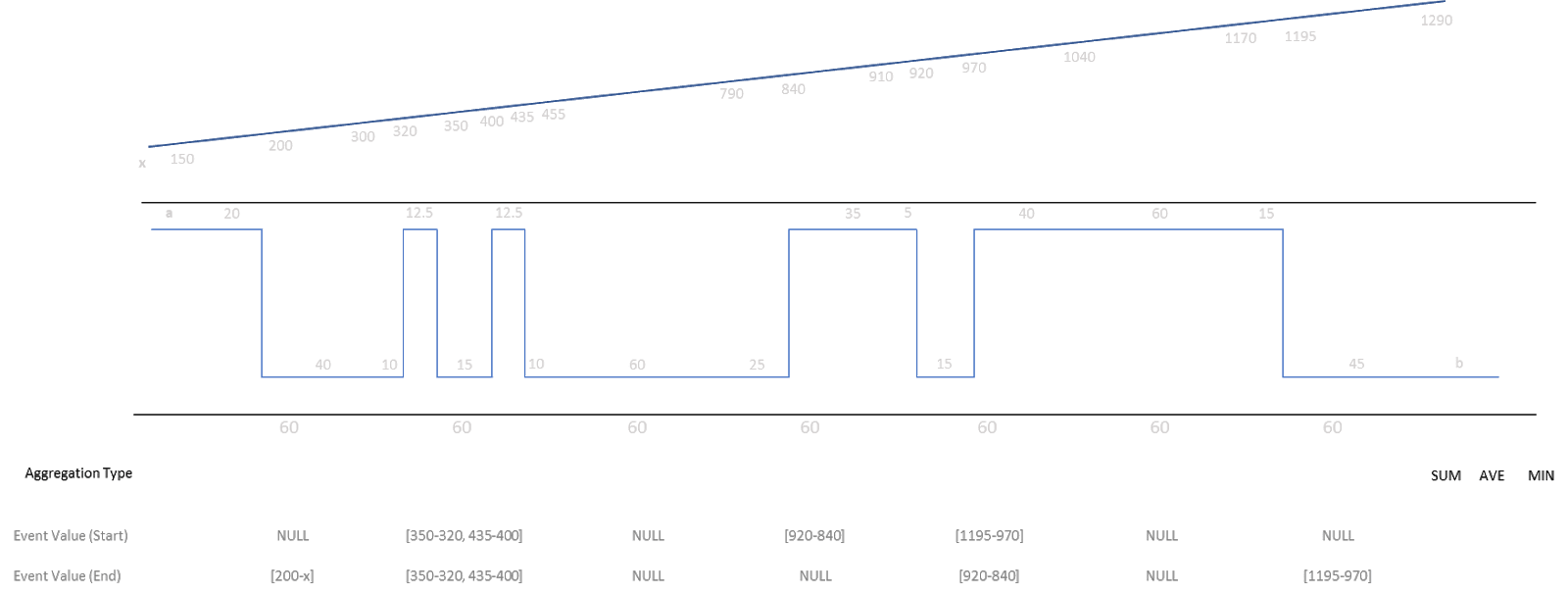The previous image helps to explain the following two event aggregation methods.

### Event Value (Start)

To configure this aggregation method, you need to configure a secondary measure that you want to perform this event aggregation method upon.

For each event that started in this interval, this returns the value of the configured measure at the start and end of each event that was triggered.

### Event Value (End)

To configure this aggregation method, you need to configure a secondary measure that you want to perform this event aggregation method upon.

For each event that ended in this interval, this returns the value of the configured measure at the start and end of each event that was triggered.